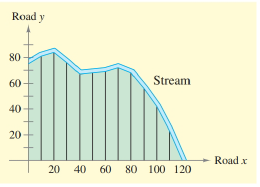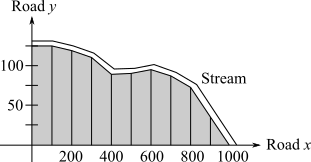Chapter 6.3, Problem 32E### Calculus: An Applied Approach (Min...

10th Edition
Ron Larson
ISBN: 9781305860919

#### Solutions

Chapter
Section### Calculus: An Applied Approach (Min...

10th Edition
Ron Larson
ISBN: 9781305860919
Textbook Problem
1 views

# Surveying In Exercises 31 and 32, use the Trapezoidal Rule to estimate the number of square feet of land in the lot, where x and y are measured in feet, as shown in the figure. The land is bounded by a stream and two straight roads. (The number of subintervals is shown in the figure.)0 10 20 30 40 50 60 y 75 81 84 76 67 68 69 X 70 80 90 100 110 120 y 72 68 56 42 23 0 Spreadsheet at LarsonAppliedCalculus.com

To determine

To calculate: The number of square feet of land in the lot where the value of x and y get measured in feet with the use of Trapezoidal Rule according to the provided figure.

The provided figure of graph with subintervals is,And Provided table is,

x0102030405060708090100110120y7581847667686972685642230

Explanation

Given Information:

The provided figure of graph with subintervals is,

And Provided table is,

x0102030405060708090100110120y7581847667686972685642230

Formula used:

Trapezoidal Rule:

If a function f is continuous on [a,b], then

abf(x)dx(ba2n)[f(x0)+2f(x1)+...+2f(xn1)+f(xn)]

Calculation:

According to provided figure the graph is divided into 12 sections, so the value of n=12.

The minimum limit of x is 0 and maximum limit of x is 120, so the value of a=0 and b=120.

And y=f(x).

Calculation by Trapezoidal Rule:

for that get the end points of subintervals are,

f(x0)=75,f(x1)=81,f(x2)=84,f(x3)=76,f(x4)=67,f(x5)=68,f(x6)=69,f

### Still sussing out bartleby?

Check out a sample textbook solution.

See a sample solution

#### The Solution to Your Study Problems

Bartleby provides explanations to thousands of textbook problems written by our experts, many with advanced degrees!

Get Started

#### Differentiate the function. y=x2+4x+3x

Single Variable Calculus: Early Transcendentals, Volume I

#### CHECK POINT 3. Evaluate the following. (a) (b) (c) (d) (e)

Mathematical Applications for the Management, Life, and Social Sciences

#### 01e2xdx= a) 2e2 1 b) `e2 1 c) 12e2 d) 12(e21)

Study Guide for Stewart's Single Variable Calculus: Early Transcendentals, 8th